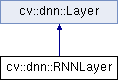OpenCV  3.2.0 Open Source Computer Vision
cv::dnn::RNNLayer Class Referenceabstract

Classical recurrent layer. More...

#include "all_layers.hpp"

Inheritance diagram for cv::dnn::RNNLayer:## Public Member Functions

void forward (std::vector< Blob *> &input, std::vector< Blob > &output)

virtual void setProduceHiddenOutput (bool produce=false)=0
If this flag is set to true then layer will produce $$h_t$$ as second output. More...

virtual void setWeights (const Blob &Wxh, const Blob &bh, const Blob &Whh, const Blob &Who, const Blob &bo)=0Public Member Functions inherited from cv::dnn::Layer
Layer ()

Layer (const LayerParams &params)
Initializes only name, type and blobs fields. More...

virtual ~Layer ()

virtual void allocate (const std::vector< Blob *> &input, std::vector< Blob > &output)=0
Allocates internal buffers and output blobs with respect to the shape of inputs. More...

void allocate (const std::vector< Blob > &inputs, std::vector< Blob > &outputs)

std::vector< Bloballocate (const std::vector< Blob > &inputs)

void forward (const std::vector< Blob > &inputs, std::vector< Blob > &outputs)

virtual int inputNameToIndex (String inputName)
Returns index of input blob into the input array. More...

virtual int outputNameToIndex (String outputName)
Returns index of output blob in output array. More...

void run (const std::vector< Blob > &inputs, std::vector< Blob > &outputs)
Allocates layer and computes output. More...

void setParamsFrom (const LayerParams &params)
Initializes only name, type and blobs fields. More...

## Static Public Member Functions

static Ptr< RNNLayercreate ()Public Attributes inherited from cv::dnn::Layer
std::vector< Blobblobs
List of learned parameters must be stored here to allow read them by using Net::getParam(). More...

String name
Name of the layer instance, can be used for logging or other internal purposes. More...

String type
Type name which was used for creating layer by layer factory. More...

## Detailed Description

Classical recurrent layer.

## § create()

 static Ptr cv::dnn::RNNLayer::create ( )
static

Creates instance of RNNLayer

## § forward()

 void cv::dnn::RNNLayer::forward ( std::vector< Blob *> & input, std::vector< Blob > & output )
virtual

Accepts two inputs $$x_t$$ and $$h_{t-1}$$ and compute two outputs $$o_t$$ and $$h_t$$.

Parameters
 input should contain packed input $$x_t$$. output should contain output $$o_t$$ (and $$h_t$$ if setProduceHiddenOutput() is set to true).

input should have shape [T, N, data_dims] where T and N is number of timestamps and number of independent samples of $$x_t$$ respectively.

output will have shape [T, N, $$N_o$$], where $$N_o$$ is number of rows in $$W_{xo}$$ matrix.

If setProduceHiddenOutput() is set to true then output will contain a Blob with shape [T, N, $$N_h$$], where $$N_h$$ is number of rows in $$W_{hh}$$ matrix.

Implements cv::dnn::Layer.

## § setProduceHiddenOutput()

 virtual void cv::dnn::RNNLayer::setProduceHiddenOutput ( bool produce = false )
pure virtual

If this flag is set to true then layer will produce $$h_t$$ as second output.

Shape of the second output is the same as first output.

## § setWeights()

 virtual void cv::dnn::RNNLayer::setWeights ( const Blob & Wxh, const Blob & bh, const Blob & Whh, const Blob & Who, const Blob & bo )
pure virtual

Setups learned weights.

Recurrent-layer behavior on each step is defined by current input $$x_t$$, previous state $$h_t$$ and learned weights as follows:

\begin{eqnarray*} h_t &= tanh&(W_{hh} h_{t-1} + W_{xh} x_t + b_h), \\ o_t &= tanh&(W_{ho} h_t + b_o), \end{eqnarray*}

Parameters
 Wxh is $$W_{xh}$$ matrix bh is $$b_{h}$$ vector Whh is $$W_{hh}$$ matrix Who is $$W_{xo}$$ matrix bo is $$b_{o}$$ vector

The documentation for this class was generated from the following file:
• /build/master-contrib_docs-lin64/opencv_contrib/modules/dnn/include/opencv2/dnn/all_layers.hpp